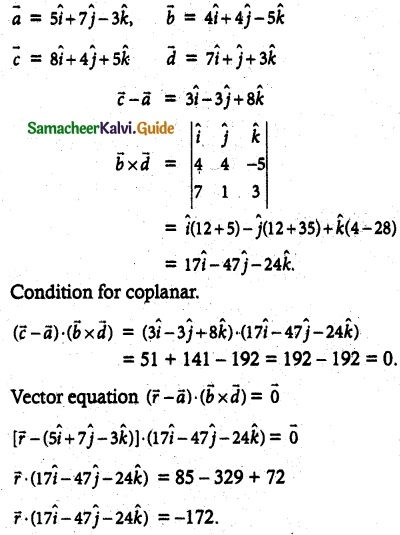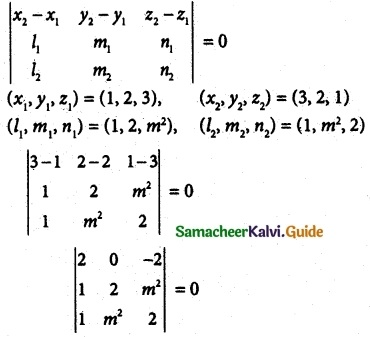Tamilnadu State Board New Syllabus Samacheer Kalvi 12th Maths Guide Pdf Chapter 6 Applications of Vector Algebra Ex 6.8 Textbook Questions and Answers, Notes.

## Tamilnadu Samacheer Kalvi 12th Maths Solutions Chapter 6 Applications of Vector Algebra Ex 6.8

Question 1.
Show that the straight lines
$$\overline { r }$$ = (5$$\hat { i }$$ + 7$$\hat { j }$$ – 3$$\hat { k }$$) + s(4$$\hat { i }$$ + 4$$\hat { j }$$ – 5$$\hat { k }$$) and
$$\overline { r }$$ = (8$$\hat { i }$$ + 4$$\hat { j }$$ + 5$$\hat { k }$$) + t(7$$\hat { i }$$ + $$\hat { j }$$ + 3$$\hat { k }$$) are coplanar. Find the vector equation of the, plane in which they lie.
Solution:Question 2.
Show that the lines $$\frac { x-2 }{ 1 }$$ = $$\frac { y-3 }{ 1 }$$ = $$\frac { z -4}{ 3 }$$ and $$\frac { x-1 }{ -3 }$$ = $$\frac { y-4 }{ 2 }$$ = $$\frac { z-5 }{ 1 }$$ are coplanar. Also, find the plane containing these lines.
Solution:Cartesian equation
x + 2y – 2z = 4
x + 2y – 2z – 4 = 0

Question 3.
If the straight lines $$\frac { x-1 }{ 1 }$$ = $$\frac { y-2 }{ 2 }$$ = $$\frac { z-3}{ m^2 }$$ and $$\frac { x-3 }{ 1 }$$ = $$\frac { y-2 }{ m^2 }$$ = $$\frac { z-1 }{ 2 }$$ are coplanar, find the distinct real values of m
Solution:2(4 – m4) – 2(m² – 2) = 0
8 – 2m4 – 2m² + 4 = 0
12 – 2m4 – 2m² = 0
(÷ -2) -6 + m4 + m² = 0
m4 + m² – 6 = 0
(m² – 2)(m² + 3) = 0
m² – 2 = 2; m² = -3 (not possible)
m² = 2
m = ±√2Question 4.
If the straight lines $$\frac { x-1 }{ 2 }$$ = $$\frac { y+1 }{ λ }$$ = $$\frac { z }{ 2 }$$ and $$\frac { x+1 }{ 5 }$$ = $$\frac { y+1 }{ 2 }$$ = $$\frac { z }{ λ }$$ are coplanar, find λ and equations of the planes containing these two lines.
Solution:
If the two lines are coplanarWhen λ = 2
(x1, y1, z1) = (1, -1, 0)
(b1, b2, b3) = (2, 2, 2)
(d1, d2, d3) = (5, 2, 2)
$$\left|\begin{array}{ccc} x-x_{1} & y-y_{1} & z-z_{1} \\ b_{1} & b_{2} & b_{3} \\ d_{1} & d_{2} & d_{3} \end{array}\right|$$ = 0
⇒ $$\left|\begin{array}{ccc} x-1 & y+1 & z-0 \\ 2 & 2 & 2 \\ 5 & 2 & 2 \end{array}\right|$$ = 0
⇒ (x – 1)(0) – (y + 1)(-6) + z(6) = 0
⇒6(y + 1) – 6z = 0
⇒ 6y + 6 – 6z = 0
⇒ y – z + 1 = 0
When λ = 2
(b1, b2, b3) = (2, -2, 2)
(d1, d2, d3) = (5, 2, -2)
⇒ $$\left|\begin{array}{ccc} x-1 & y+1 & z-0 \\ 2 & -2 & 2 \\ 5 & 2 & -2 \end{array}\right|$$ = 0
⇒ (x – 1)(0) – (y + 1)(-14) + z(4 + 10) = 0
⇒ 14(y + 1) + 14z = 0
⇒ 14y + 14 + 14z = 0
⇒ y + z + 1 = 0